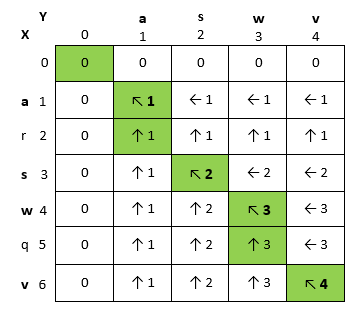# Find longest common sub-sequence from two given strings

 Category: Data Structure And Algorithms Tags:Longest Common Subsequence Problem Code Files

## Introduction

Sometimes we are given with two strings and we need to find longest common sub-sequence in these to compare how closely these are related to each other, like we have two subsets S1 = {a, r, s, w, q, v} and other one S2 = {a, s, w, v} so we can see elements of S2 appears in S1 with increasing order {1, 3, 4, 6} so we can say S2 itself is common sub-sequence of S1. Simply we have to find matching characters in increasing order in given strings.

## Implementation

In this section we are going to solve this problem using dynamic programming and we have already discussed what is dynamic programming in previous article. Suppose given strings X and Y having lengths m and n respectively. If we want to solve this problem comparing each character of X to all characters of Y then we are going to have m.n comparison and complexity of algorithm will be Θ(m.n). To solve this problem we have to consider 2 cases:

1. If Xm == Yn then this is a match and solve sub-sequence of Xm-1 and Yn-1
2. If Xm != Yn then:
1. Solve sub-sequence of Xm-1 and Y
2. Solve sub-sequence of X and Yn-1

Above cases you can understand while taking example of s1 and s2 from introduction section, if we start comparing strings from end then we will see:

1. S1 equals to S2 => is a match and solve S1(1, 5) and S2(1, 3) in next step
2. S1 doesn’t equal to S2 =>
1. Solve S1(1, 4) and S2(1, 3)
2. solve S1(1, 5) and S2(1, 2)

Using dynamic programming we can easily track how many matches we have found already at any point. To solve this pro-grammatically we need two matrices one to keep track of counts of matches at every index and other one to keep directions from one match to another match(that you can see arrows in diagram below), below is the structure of class:

```public class LCS
{
string S1, S2;  //Strings to match
int m, n;   //Lengths of S1 and S2
char[,] b;  //Stores direction
int[,] c;   //Stores count
public LCS(string s1, string s2)
{
this.S1 = s1;
this.S2 = s2;
m = s1.Length;
n = s2.Length;
b = new char[m, n];
c = new int[m + 1, n + 1];
}

public int FindLCS(){...}
private void PrintLCS(char[,] b, string s1, int i, int j){...}
}
```

First we will see FindLCS method which actually creates solutions:

```public int FindLCS()
{
for (int i = 1; i <= m; i++) //1 to m
for (int j = 1; j <= n; j++) //1 to n
{
if (S1[i - 1] == S2[j - 1]) //Xm == Yn
{
c[i, j] = c[i - 1, j - 1] + 1;
b[i - 1, j - 1] = 'd';
}
else if (c[i - 1, j] >= c[i, j - 1])    //compare Xm-1 to Yn
{
c[i, j] = c[i - 1, j];
b[i - 1, j - 1] = 'u';
}
else
{
c[i, j] = c[i, j - 1];
b[i - 1, j - 1] = 'l';
}
}

PrintLCS(b, S1, m - 1, n - 1);
return c[m, n];
}
```

Above we can see we are storing counts at every row and directions using array “b” where ‘d’ means diagonal, ‘u’ means up and ‘l’ means left. If we find a match we have to increment count from previous sub-problem and mark direction as ‘d’ else we will mark directions up or left. Count actually shows how many matches has been found till current index and direction shows way of matching sequences.Above marked in darks are matches, last index c[m, n] is the total matches and green path directs matches sequence. To print this we need to implement PrintLCS method:

```private void PrintLCS(char[,] b, string s1, int i, int j)
{
if (i == -1 || j == -1)
return;

if (b[i, j] == 'd')
{
PrintLCS(b, s1, i - 1, j - 1);
Console.WriteLine(s1[i]);
}
else if (b[i, j] == 'u')
{
PrintLCS(b, s1, i - 1, j);
}
else
{
PrintLCS(b, s1, i, j - 1);
}
}```

Now we can execute this code from our Main method:

```static void Main(string[] args)
{
LCS lcs = new LCS("arswqv", "aswv");
int count = lcs.FindLCS();
Console.WriteLine("Total Matches: " +count);    Console.ReadLine();
}
```

Output:

a

s

w

v

Total Matches: 4

## Analysis

Above we could see we need m.n loop executions to solve this problem and complexity of this algorithm will be same Θ(m.n).

 Like0 People
Last modified on 31 October 2018Nikhil Joshi Ceo & Founder at Dotnetlovers Atricles: 132 Questions: 9 Given Best Solutions: 9 *

## Comments:

No Comments Yet

You are not loggedin, please login or signup to add comments:

## Existing User

Login via:## New Userx